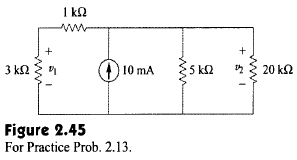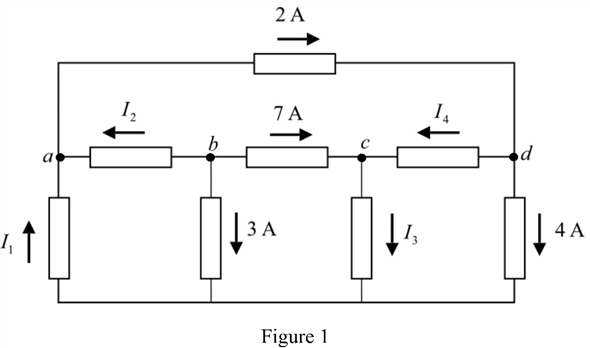Problem

# For the circuit shown in Fig. 2.45, find: (a) v1and v2,(b) the power dissipated in the 3-k...

For the circuit shown in Fig. 2.45, find: (a) v1and v2,(b) the power dissipated in the 3-kfl and 20-kfl resistors, and (c) the power supplied by the current source.Answer:(a) 15V, 20V, (b) 75 mW, 20 mW, (c) 200 mW.

#### Step-by-Step Solution

Solution 1

Refer to Figure 2.77 in the textbook.

Redraw the circuit with node notations, as shown in Figure 1.Apply Kirchhoff's current law at node $$d$$.

\begin{aligned} &2-I_{4}-4=0 \\ &I_{4}=-4+2 \\ &=-2 \mathrm{~A} \end{aligned}

Hence, the current $$I_{4}$$ is, $$-2 \mathrm{~A}$$.

Apply Kirchhoff's current law at node $$c$$.

$$I_{4}-I_{3}+7=0$$

Substitute $$-2$$ for $$I_{4}$$.

\begin{aligned} &-2-I_{3}+7=0 \\ &I_{3}=5 \mathrm{~A} \end{aligned}

Hence, the current $$I_{3}$$ is, $$5 \mathrm{~A}$$.

Apply Kirchhoff's current law at node $$b$$.

\begin{aligned} &-3-I_{2}-7=0 \\ &I_{2}=-10 \mathrm{~A} \end{aligned}

Hence, the current $$I_{2}$$ is, $$-10 \mathrm{~A}$$.

Apply Kirchhoff's current law at node a.

$$I_{1}+I_{2}-2=0$$

Substitute $$-10$$ for $$I_{2}$$

\begin{aligned} &I_{1}-10-2=0 \\ &I_{1}=12 \mathrm{~A} \end{aligned}

Hence, the current $$I_{1}$$ is, $$12 \mathrm{~A}$$.